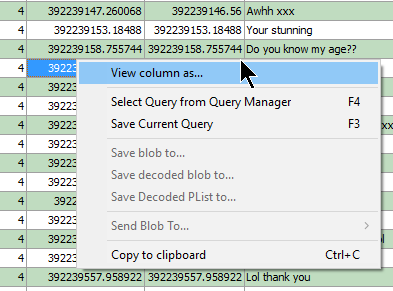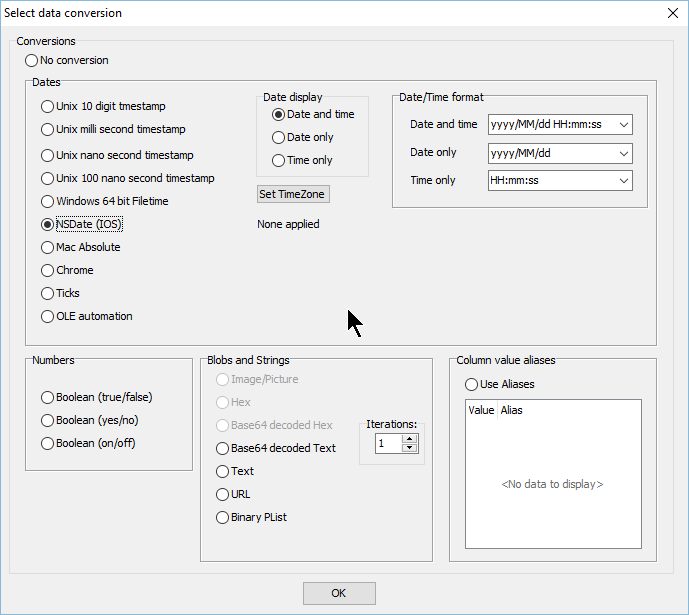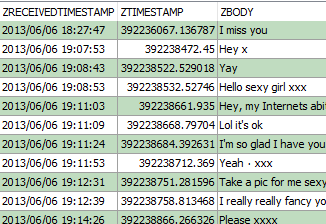The Forensic Browser for SQLite incorporates features such that you can right click on a numeric date column and have the Browser convert a number to one of the supported date formats, applying a timezone offset as required.

The process is simply – right-click the required column and choose “View column as…”.Select the date format that you believe the column is saved as, in this case I recognise this as an IOS NSDate format:And it’s that simple…So how can we validate the conversion done by the Forensic Browser? The method I choose is to use the SQLite built in functions within the Forensic Browser, as follows.

We can do this by simply having two copies of the column that we are converting within the same query an dthe using the SQLite DateTime fucntion to convert and display the date, the SQL is as follows:

SELECT ZKIKMESSAGE.ZTIMESTAMP,
DateTime(ZKIKMESSAGE.ZTIMESTAMP + 978307200, ‘unixepoch’) AS SQLiteConvertedTime,
ZKIKMESSAGE.ZBODY
FROM ZKIKMESSAGE

* The first line selects the ZTIMESTAMP column (and we apply an NSDate conversion using the Browsers built in features as above)
* The second line selects the ZTIMESTAMP column again, does some internal SQLite stuff – more shortly) and displyas it in a column with the alias SQLiteConvertedTime
* the third column adds the ZBODY field just so we can reference teach row to the screen shot above
* and finally the fourth row specifies the table we are using

The results of this query are as below:As you can see the timestamps are the same and the two date columns compare as expected. The beauty of this method is that you do not need to leave the Browser but you are directly calling a function (DateTime) from the SQLite3.dll and thus bypassing the Browser for an independant date validation.

So how does it work?

The Browser takes the numeric value from the ZTIMESTAMP column adds 978307200 to it and then tells SQLite to convert it to a human-readable string and treating the new number as a unixepoch date.

DateTime(ZKIKMESSAGE.ZTIMESTAMP + 978307200, ‘unixepoch’)

The NSDate format records the number of seconds since 1/1/2001 and the unix date format records the number of seconds since 1/1/1970. 978307200 is the number of seconds between the two dates (often referred to as the Delta), this figure is added to adjust the NSDate value to a unix value.

The relevant web page for the DateTime function is as follows:

### Similarly, other supported date formats can be validated as follows:

Unix 10 digit dates

The number of seconds since 01/01/1970

Unix dates can be validated simply by using the date “as is”, i.e. there is no need to apply a delta

The SQL query is:

DateTime(numeric_time, ‘unixepoch’) AS Converted,

Unix milliseconds (13 digit) dates

The number of milliseconds since 01/01/1970

as above but convert to seconds by dividing by 1,000

The SQL query is:

DateTime((numeric_time / 1000, ‘unixepoch’) AS Converted,

Unix nano second and 100 nano second dates

These are the same as above but use 1,000,000,000 and 10,000,000 respectively as the divisor.

Chrome/webkit time

This is the number of microsecond intervals since 01/01/1601

The Delta (difference) between 01/01/1601 and 01/01/1970 is 11644473600 seconds, so first convert the Chrome date from microseconds to seconds by dividing by 1,000,000 then just take away the delta (we take it away because the chrome epoch date [01/01/1601] is older than the unix epoch)

The SQL query is:

DateTime((numeric_time / 1000000) – 11644473600, ‘unixepoch’) AS Converted

Windows 64 bit filetime

This is very similar to the Chrome date except the interval is the number of 100 nanoseconds since 01/01/1601. Therefore instead of dividing by 1,000,000, we need to divide by 10,000,000

The SQL query is:

DateTime((numeric_time / 10000000) – 11644473600, ‘unixepoch’) AS Converted

NSDate (IOS)

Records the number of seconds since 01/01/2001

The SQL query is:

DateTime(numeric_time + 978307200, ‘unixepoch’) AS Converted

Mac

Records the number of seconds since 01/01/1904

The SQL query is:

DateTime(numeric_time – 2082844800, ‘unixepoch’) AS Converted

OLE Automation

Records the number of days and fractions of a day since 30/12/1899

Using an “example date” of 42439.766146 the query we want is:

SELECT DateTime(42439.766146 * 86400 – 2209161600, ‘unixepoch’) AS Converted

i.e. convert the fractional day portion into seconds by multiplying by the seconds in a day.